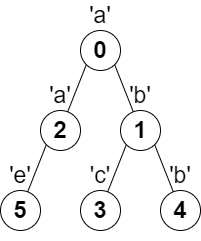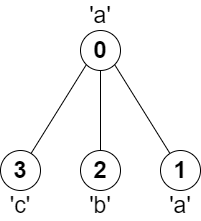##### Welcome to Subscribe On Youtube

Formatted question description: https://leetcode.ca/all/2246.html

# 2246. Longest Path With Different Adjacent Characters

• Difficulty: Hard.
• Related Topics: Array, String, Tree, Depth-First Search, Graph, Topological Sort.
• Similar Questions: Diameter of Binary Tree, Longest Univalue Path, Choose Edges to Maximize Score in a Tree.

## Problem

You are given a tree (i.e. a connected, undirected graph that has no cycles) rooted at node 0 consisting of n nodes numbered from 0 to n - 1. The tree is represented by a 0-indexed array parent of size n, where parent[i] is the parent of node i. Since node 0 is the root, parent == -1.

You are also given a string s of length n, where s[i] is the character assigned to node i.

Return the length of the **longest path in the tree such that no pair of adjacent nodes on the path have the same character assigned to them.**

Example 1:Input: parent = [-1,0,0,1,1,2], s = "abacbe"
Output: 3
Explanation: The longest path where each two adjacent nodes have different characters in the tree is the path: 0 -> 1 -> 3. The length of this path is 3, so 3 is returned.
It can be proven that there is no longer path that satisfies the conditions.


Example 2:Input: parent = [-1,0,0,0], s = "aabc"
Output: 3
Explanation: The longest path where each two adjacent nodes have different characters is the path: 2 -> 0 -> 3. The length of this path is 3, so 3 is returned.


Constraints:

• n == parent.length == s.length

• 1 <= n <= 105

• 0 <= parent[i] <= n - 1 for all i >= 1

• parent == -1

• parent represents a valid tree.

• s consists of only lowercase English letters.

## Solution

• class Solution {
public int longestPath(int[] parent, String s) {
// for first max length
int[] first = new int[s.length()];
Arrays.fill(first, 0);
// for second max length
int[] second = new int[s.length()];
Arrays.fill(second, 0);
// for number of children for this node
int[] children = new int[s.length()];
Arrays.fill(children, 0);
for (int i = 1; i != s.length(); i++) {
// calculate all children for each node
children[parent[i]]++;
}
// for traversal from leafs to root
// put all leafs
for (int i = 1; i != s.length(); i++) {
if (children[i] == 0) {
}
}
// traversal from leafs to root
while (!st.isEmpty()) {
// fetch current node
int i = st.pollLast();
// if we in root - ignore it
if (i == 0) {
continue;
}
if (--children[parent[i]] == 0) {
// decrease number of children by parent node and if number of children
}
// is equal 0 - our parent became a leaf
// if letters isn't equal
if (s.charAt(parent[i]) != s.charAt(i)) {
// fetch maximal path from node
int maxi = 1 + Math.max(first[i], second[i]);
// and update maximal first and second path from parent
if (maxi >= first[parent[i]]) {
second[parent[i]] = first[parent[i]];
first[parent[i]] = maxi;
} else if (second[parent[i]] < maxi) {
second[parent[i]] = maxi;
}
}
}
int ans = 0;
for (int i = 0; i != s.length(); i++) {
ans = Math.max(ans, first[i] + second[i]);
}
return ans + 1;
}
}

• # 2246. Longest Path With Different Adjacent Characters

class Solution:
def longestPath(self, parent: List[int], s: str) -> int:
n = len(parent)
graph = defaultdict(list)
res = 0

for x, y in enumerate(parent):
if y == -1 or s[x] == s[y]: continue

graph[y].append(x)
graph[x].append(y)

@cache
def go(node, prev):
nonlocal res

path = 

for nei in graph[node]:
if nei != prev:
path.append(go(nei, node) + 1)

path.sort(reverse = 1)

if len(path) == 1:
res = max(res, path)
else:
res = max(res, path + path - 1)

return path

for i in range(n):
go(i, -1)

return res


• function longestPath(parent: number[], s: string): number {
const n = parent.length;
let graph = Array.from({ length: n }, v => []);
for (let i = 1; i < n; i++) {
graph[parent[i]].push(i);
}
let ans = 0;
function dfs(x: number): number {
let maxLen = 0;
for (let y of graph[x]) {
let len = dfs(y) + 1;
if (s.charAt(x) !== s.charAt(y)) {
ans = Math.max(maxLen + len, ans);
maxLen = Math.max(len, maxLen);
}
}
return maxLen;
}
dfs(0);
return ans + 1;
}


• class Solution {
public:
int longestPath(vector<int>& parent, string s) {
int n = parent.size();
vector<int> g[n];
for (int i = 1; i < n; ++i) {
g[parent[i]].push_back(i);
}
int ans = 0;
function<int(int)> dfs = [&](int i) -> int {
int mx = 0;
for (int j : g[i]) {
int x = dfs(j) + 1;
if (s[i] != s[j]) {
ans = max(ans, mx + x);
mx = max(mx, x);
}
}
return mx;
};
dfs(0);
return ans + 1;
}
};

• func longestPath(parent []int, s string) int {
n := len(parent)
g := make([][]int, n)
for i := 1; i < n; i++ {
g[parent[i]] = append(g[parent[i]], i)
}
ans := 0
var dfs func(int) int
dfs = func(i int) int {
mx := 0
for _, j := range g[i] {
x := dfs(j) + 1
if s[i] != s[j] {
ans = max(ans, x+mx)
mx = max(mx, x)
}
}
return mx
}
dfs(0)
return ans + 1
}

func max(a, b int) int {
if a > b {
return a
}
return b
}


Explain:

nope.

Complexity:

• Time complexity : O(n).
• Space complexity : O(n).